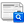Transformation of the generalized chaotic system into canonical form

(1) * Roman Voliansky(Dniprovsky State Technical University, Ukraine)
*corresponding authorAbstract

The paper deals with the developing of the numerical algorithms for transformation of generalized chaotic system into canonical form. Such transformation allows us to simplify control algorithm for chaotic system. These algorithms are defined by using Lie derivatives for output variable and solution of nonlinear equations. Usage of proposed algorithm is one of the ways for discovering of new chaotic attractors. These attractors can be obtained by transformation of known chaotic systems into various state spaces. Transformed attractors depend on both parameters of chaotic system and sample time of its discrete model.

Keywords

Chaos; Dynamical system; Differential equations; Generalized chaotic system; Nonlinear coordinate transformation

DOI

https://doi.org/10.26555/ijain.v3i3.113

Article metrics

Abstract views : 1412 | PDF views : 434

CiteHow to cite item

Full TextDownload

References

C. K. Volos, I. M. Kyprianidis, and I. N. Stouboulos, “A chaotic path planning generator for autonomous mobile robots,” Rob. Auton. Syst., vol. 60, no. 4, pp. 651–656, 2012.

R. Voliansky and A. Sadovoy, “Chua’s circuits synchronization as inverse dynamic’s problem solution,” in Problems of Infocommunications Science and Technology (PIC S&T), 2016 Third International Scientific-Practical Conference, 2016, pp. 171–172.

L. M. Pecora and T. L. Carroll, “Synchronization in chaotic systems,” Phys. Rev. Lett., vol. 64, no. 8, p. 821, 1990.

S. Kim, Y. Kim, J. Lee, and H. S. Kim, “Identifying and Evaluating Chaotic Behavior in Hydro-Meteorological Processes,” Adv. Meteorol., vol. 2015, 2015.

O. Bajo-Rubio, F. Fernandez-Rodriguez, and S. Sosvilla-Rivero, “Chaotic behaviour in exchange-rate series: First results for the Peseta—US dollar case,” Econ. Lett., vol. 39, no. 2, pp. 207–211, 1992.

P. Zhou, K. Huang, and C. Yang, “A fractional-order chaotic system with an infinite number of equilibrium points,” Discret. Dyn. Nat. Soc., vol. 2013, 2013.

S. Vaidyanathan, “A novel 3-D jerk chaotic system with three quadratic nonlinearities and its adaptive control,” Arch. Control Sci., vol. 26, no. 1, pp. 19–47, 2016.

C. Yin, S. Zhong, and W. Chen, “Design of sliding mode controller for a class of fractional-order chaotic systems,” Commun. Nonlinear Sci. Numer. Simul., vol. 17, no. 1, pp. 356–366, 2012.

T.-C. Lin and C.-H. Kuo, “H∞ synchronization of uncertain fractional order chaotic systems: adaptive fuzzy approach,” ISA Trans., vol. 50, no. 4, pp. 548–556, 2011.

A. Isidori, Nonlinear control systems. Springer Science & Business Media, 2013.

S. ČELIKOVSKÝ and G. Chen, “On a generalized Lorenz canonical form of chaotic systems,” Int. J. Bifurc. Chaos, vol. 12, no. 8, pp. 1789–1812, 2002.

T. Zhou, G. Chen, and S. ČelikovskÝ, “Ši’lnikov chaos in the generalized Lorenz canonical form of dynamical systems,” Nonlinear Dyn., vol. 39, no. 4, pp. 319–334, 2005.

M. Reuter, S. Biasotti, D. Giorgi, G. Patanè, and M. Spagnuolo, “Discrete Laplace--Beltrami operators for shape analysis and segmentation,” Comput. Graph., vol. 33, no. 3, pp. 381–390, 2009.

E. Süli and D. F. Mayers, An introduction to numerical analysis. Cambridge university press, 2003.

A. N. Pchelintsev, “Numerical and physical modeling of the dynamics of the Lorenz system,” Numer. Anal. Appl., vol. 7, no. 2, pp. 159–167, 2014.International Journal of Advances in Intelligent InformaticsThis work is licensed under a Creative Commons Attribution-ShareAlike 4.0